# How to Use the IFERROR Excel FunctionClaudia Buckley#### Join the Excel conversation on Slack

Ask a question or join the conversation for all things Excel on our Slack channel.

IFERROR in Excel is a really useful logical function that tells Excel what to do if the formula that was entered returns an error response

It has two elements — the argument that is to be evaluated, and the value to be returned if the result of that argument is an error. Both arguments are required.

The syntax of the IFERROR Excel function is:

``=IFERROR(value, value_if_error)``
• Value is the argument, calculation, or cell reference which is checked for an error.
• Value_if_error is a customized value or calculation to return if the first argument is evaluated to be an error.

Excel considers the following responses as errors:

• #N/A
• #VALUE!
• #REF!
• #DIV/0!
• #NUM!
• #NAME?
• #NULL!

Below is a simple example.

Use this free IFERROR Excel file to practice along with the tutorial.

## If error, then blank

To find out the unit cost of each item on the spreadsheet below, we would simply divide the price by the number that comes in each crate.

``=B2/C2``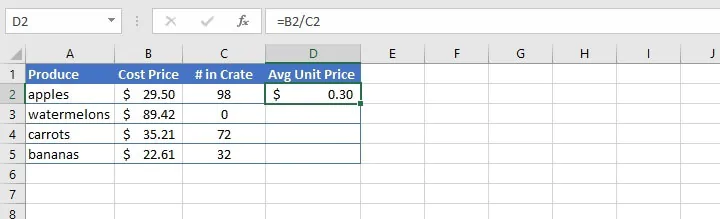This formula results in an error for the second item, since values divided by zero result in a #DIV/0! error.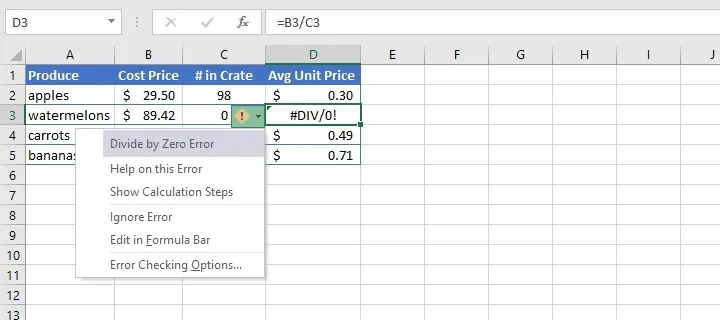IFERROR allows us to state the value that Excel should return instead of displaying the error message.

``=IFERROR(C3/B3,"")``

Typing a pair of double empty quotes tells Excel to display a blank cell if the result of the formula is an error.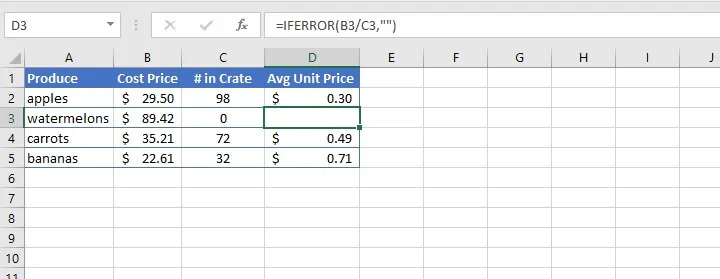## If error, then perform a calculation

Another option for IFERROR’s value_if_error is to perform another calculation if the first formula results in an error. Because of how simple it is to understand and use the IFERROR Excel function, it is quite easy to combine it with other formulas to get clean-looking results.

The spreadsheet below adds employees’ hours worked and paid time off, then multiplies that total by their hourly rate to calculate the gross monthly salary. This results in a #VALUE! error for rows that contain text values.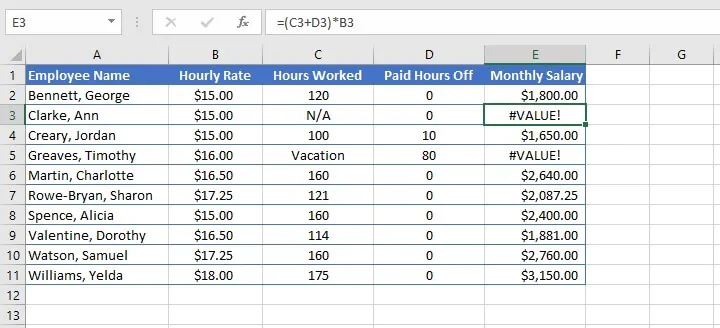The value_if_error argument within the IFERROR function can be used to perform an alternative calculation in this instance.

``=IFERROR((C3+D3)*B3,D3*B3)``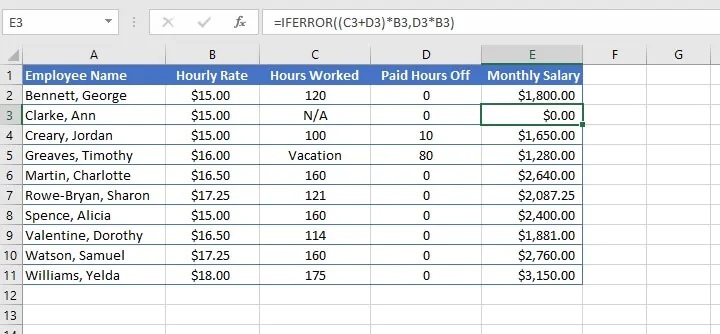As shown in the above image, the alternative calculation is used to omit the column which causes the error.

## If error, then show a message

IFERROR is often used with lookup functions, especially in cases where the end user may not be the owner of the worksheet, or may not be familiar with Excel formulas. We usually want to communicate clearly if the input results in an unexpected result, so IFERROR offers a great solution.

In the example below, when we enter an ID number in cell E2, we expect the VLOOKUP formula in E3 to look up the value in the dataset A2 to B7, and return the employee’s name.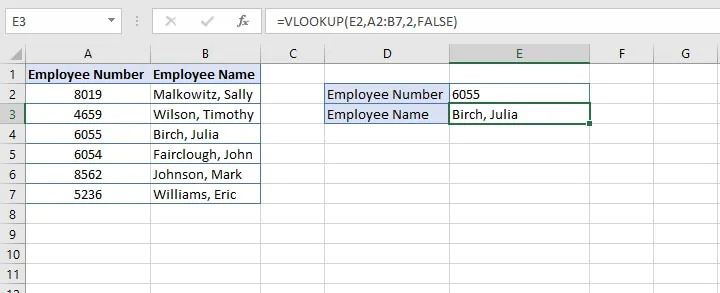However, if an incorrect ID number is entered in cell E2, an error message is displayed which may not be readily understood.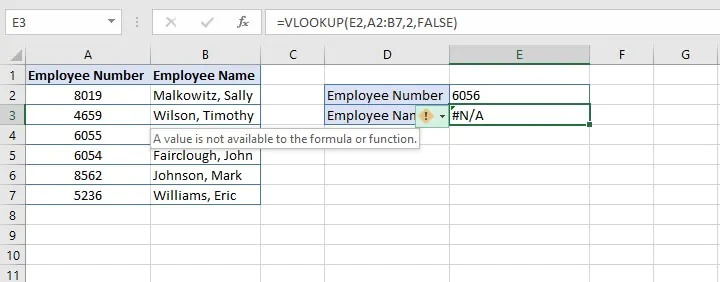The return value (#N/A) is cryptic at best. And Excel’s trace formula error message — “A value is not available to the formula or function” — isn’t particularly helpful either.

We can use the IFERROR Excel function to create our own customized message. We already have in place the formula that we want Excel to evaluate, which will now become the first argument of the IFERROR function.

The second argument will be the message we want to display if that formula results in an error. The message must be entered within double quotes.

``=IFERROR(VLOOKUP(E2,A2:B7,2,FALSE),"ID Number not found")``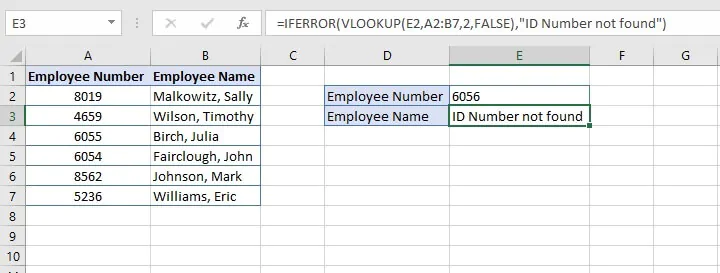Note that IFERROR treats all the previously-mentioned error responses the same — i.e., whether Excel’s error response is #N/A, #NAME?, #DIV/0! etc., IFERROR has no way of returning one response for one type of error and another response for a different type of error.

## IFERROR vs IFNA

If you want to return a customized response only for #N/A errors, you should consider using the IFNA function instead. The IFNA function allows the customization of Excel’s response only when an argument results in a #N/A error, ignoring all other error types.

In the following example, the row with the input cell (E2) was mistakenly deleted, which would normally result in a #REF! error since the original cell reference is no longer valid.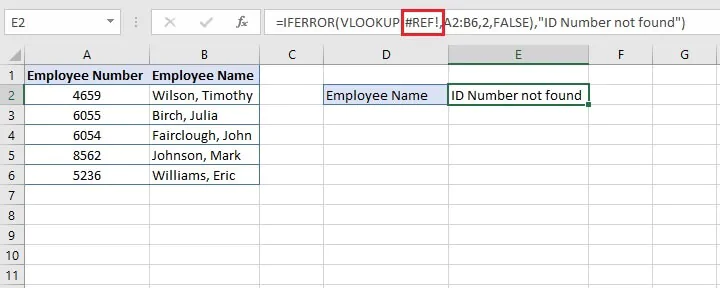However, IFERROR is unable to distinguish between the different types of errors and still returns the customized message — ID Number not found.

Using the IFNA function instead maintains the #REF! output, and we can click on the trace formula error message to learn why: “Moving or deleting cells caused an invalid cell reference or function is returning reference error.”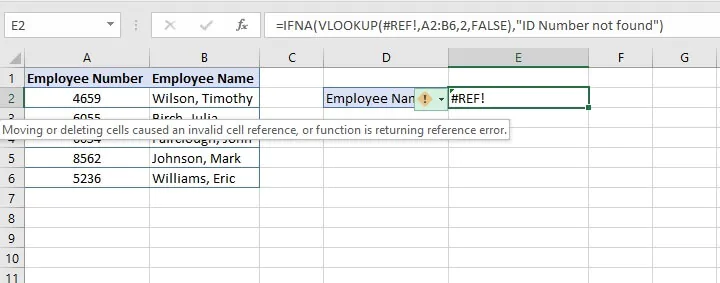It may therefore be preferable to use IFNA over IFERROR in some settings, to make troubleshooting of errors a more straightforward task.

## IFERROR vs IF ISERROR

Not to be confused with IFERROR is the ISERROR function, which just identifies whether a formula will result in an error or not by returning TRUE or FALSE.

Functions that return TRUE or FALSE are categorized under the “Information” section of the Excel Function Library. ISERROR is an older function and was frequently nested with the IF function to do what IFERROR does now.

• The ISERROR function consists of only one argument, which is determined to be true or false. The syntax is:
``=ISERROR(value)``
• The IF function has three arguments and tells Excel what to do if a certain condition is met, and what to do if that condition is not met. The syntax of the IF function is:
``=IF(logical_test,[value_if_true, value_if_false])``

ISERROR can be nested as the logical test of the IF function, followed by instructions telling Excel what to do if that evaluation does result in an error, and what to do if it does not.

Interestingly,

``=IF(ISERROR(B3/B2), “Item out of stock”, B3/B2)``

gives the same result as

``=IFERROR(B3/B2, “Item out of stock”)``

Aside from being a shorter formula, the IFERROR version performs the calculation only once, resulting in a spreadsheet that’s potentially faster than the IF ISERROR combination.

One advantage of IF ISERROR, however, is that it allows the flexibility to return text other than TRUE or FALSE rather than performing a calculation.

``=IF(ISERROR(B3/C3),"Out of stock","Available")``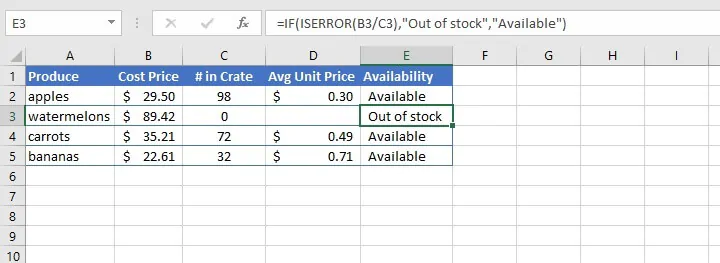## Sequential VLOOKUPs

An advanced but quite useful option for IFERROR’s value_if_error is to perform another VLOOKUP if the first VLOOKUP results in an error. This is done by nesting one lookup formula as the argument of another and is sometimes called a sequential lookup.

In the following example, we want VLOOKUP to look up the value entered in cell H2 by searching two separate arrays — first, the A3 to B8 array, then the D3 to E7 array.

``=IFERROR(VLOOKUP(H2,A3:B8,2,FALSE),(VLOOKUP(H2,D3:E7,2,FALSE)))``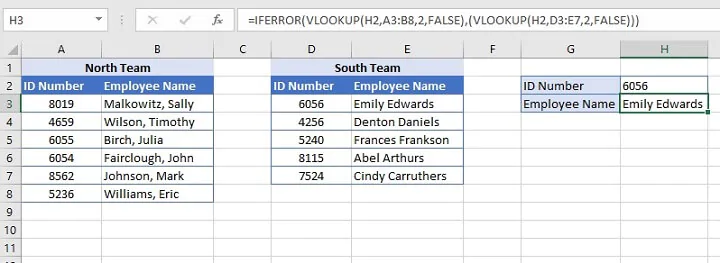The second VLOOKUP formula becomes the value_if_error argument of the IFERROR formula.

Of course, it makes sense to create a value_if_error if the second lookup also fails, by also nesting the second VLOOKUP with an IFERROR formula.

``=IFERROR(VLOOKUP(H2,A3:B8,2,FALSE),IFERROR(VLOOKUP(H2,D3:E7,2,FALSE),"ID Number not found"))``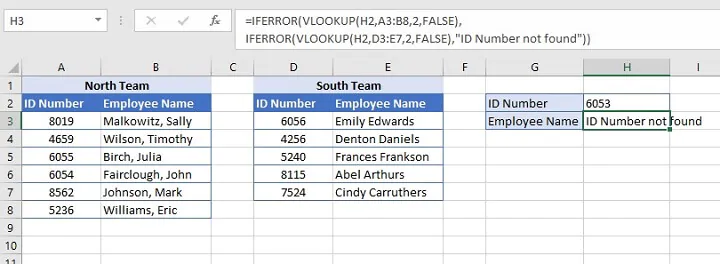A maximum of 64 IF functions may be nested in a single Excel formula, and VLOOKUPS can be linked to data in another worksheet or even another open workbook. This makes their combination quite powerful.

So, what do you think? Will you be using more of the IFERROR Excel function now that you know how it works?

Take advantage of even more Excel tools by learning Excel through our range of Excel courses. You can start with our free Excel in an Hour course and then take things to the next level with our Excel Basic and Advanced course.

Start learning formulas, functions, and time-saving hacks today with this free course!#### Join the Excel conversation on Slack

Ask a question or join the conversation for all things Excel on our Slack channel.Claudia Buckley

Claudia is a project manager and business skills instructor at GoSkills. In her spare time, she reads mystery novels and does genealogy research.